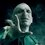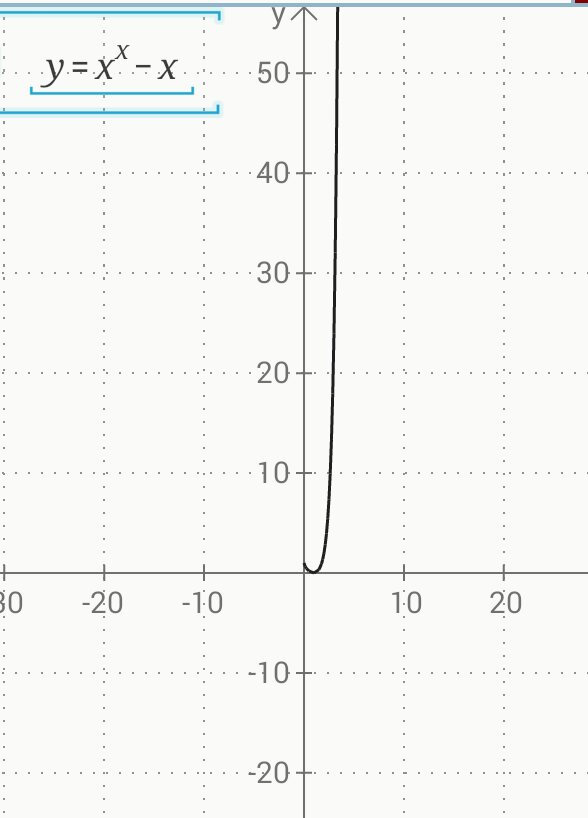# Persisting doubts

I am still confused with the problem Simply Exponential $\quad \quad \quad \quad { x }^{ x }=x\\ \Longrightarrow x={ x }^{ 1/x }\\ \Longrightarrow { x }^{ x }={ x }^{ 1/x }\\ \Longrightarrow x\ln { x } =\frac { 1 }{ x } \ln { x } \\ \Longrightarrow \ln { x } (\frac { { x }^{ 2 }-1 }{ x } )=0\ \\ \Longrightarrow x=\pm 1(Correct \quad answer)$ Now,since we have included a step $$x\ln { x } =\frac { 1 }{ x } \ln { x }$$ in our solution,according to the domain of the logarithmic function, x should be positive,thus omitting -1.Then why -1(as we cannot take log of a negative number)?

Then, I am confused that the given expression(actually an equation) is a function or not. I mean, if it is given that $f\left( x \right) ={ x }^{ x }-x$ and then we are asked to find the roots or zeroes of the function, then the answer will be only +1 or still be both+1 & -1?(I think that it should be only +1 as the domain of $x^{x}$ is $x>0$ )

Also, I don't know why, but is the second step correct and safe?

Please clear my doubts,and please provide any kind of guidance wherever you think is needed. ThanksNote by Abhijeet Verma
5 years, 3 months ago

This discussion board is a place to discuss our Daily Challenges and the math and science related to those challenges. Explanations are more than just a solution — they should explain the steps and thinking strategies that you used to obtain the solution. Comments should further the discussion of math and science.

When posting on Brilliant:

• Use the emojis to react to an explanation, whether you're congratulating a job well done , or just really confused .
• Ask specific questions about the challenge or the steps in somebody's explanation. Well-posed questions can add a lot to the discussion, but posting "I don't understand!" doesn't help anyone.
• Try to contribute something new to the discussion, whether it is an extension, generalization or other idea related to the challenge.

MarkdownAppears as
*italics* or _italics_ italics
**bold** or __bold__ bold
- bulleted- list
• bulleted
• list
1. numbered2. list
1. numbered
2. list
Note: you must add a full line of space before and after lists for them to show up correctly
paragraph 1paragraph 2

paragraph 1

paragraph 2

[example link](https://brilliant.org)example link
> This is a quote
This is a quote
    # I indented these lines
# 4 spaces, and now they show
# up as a code block.

print "hello world"
# I indented these lines
# 4 spaces, and now they show
# up as a code block.

print "hello world"
MathAppears as
Remember to wrap math in $$ ... $$ or $ ... $ to ensure proper formatting.
2 \times 3 $2 \times 3$
2^{34} $2^{34}$
a_{i-1} $a_{i-1}$
\frac{2}{3} $\frac{2}{3}$
\sqrt{2} $\sqrt{2}$
\sum_{i=1}^3 $\sum_{i=1}^3$
\sin \theta $\sin \theta$
\boxed{123} $\boxed{123}$

Sort by:

See Ivan's solution, which avoids the numerous issues that you have raised.

Whenever we manipulate an equation, we have to be careful about the steps that we're taking, and check to see if we're introducing extraneous solutions, or losing actual solutions. For example, by taking logs, we need to assume that $x > 0$.

Staff - 5 years, 3 months ago- 5 years, 3 months ago

I think $x = +1$ is the only correct solution because domain of our function is $x > 0$

- 5 years, 3 months ago

It is not stated that the domain is $x > 0$. It asks for "distinct real solutions".

Staff - 5 years, 3 months ago

If some function has domain $x > 0$ ,then how could it have a negative solution?
Before finding the solution of some function, don't we first check its domain?

- 5 years, 3 months ago

I think that we're dealing with two distinct questions. If we are just looking for solutions to the equation $x^{x} = x$ then both $1$ and $-1$ are valid solutions, and thus the answer to the question Abhijeet has linked to is $2.$

If, however, we were asked for the number of roots of the function $f(x) = x^{x} - x,$ then we would have be mindful of the domain. If we establish the domain as $x \gt 0$ then there would be only one valid root, namely $x = 1.$ If we were to also include the set of all negative numbers in the domain then $x = -1$ would also be a valid root. The more "standard" choice of domain would be $x \gt 0,$ though, since $f(x)$ would then be continuous and differentiable over all the domain.

- 5 years, 3 months ago

Thanks for inserting the graph. Yes, analytically,only +1 is the solution or zero of the function(still, -1 is confusing as $f(-1)=0$),as mentioned by Brian Sir, but -1 is a valid solution of the equation $x^{x}=x$ [if not considering it a function],which we get by hit & trial.

- 5 years, 3 months ago

From where do you get this question?

- 5 years, 3 months ago

I have mentioned the link in the note itself.

- 5 years, 3 months ago

There can be a big debate on this self-contradicting question.

- 5 years, 3 months ago

What surprised me was that the all the reports were marked as resolved.

- 5 years, 3 months ago

The reports were resolved because they are not valid. The main reasons were:

• They did not justify that there is only 1 solution.
• They did not read the question and see that it asks for the total number of solutions (esp those that say $2^ 2 \neq 2$).
• Claiming that -1 is not a solution, when it does satisfy the conditions.
• Their steps were invalid.

Staff - 5 years, 3 months ago

Surely sir, I meant that seeing all reports as resolved made me think again and then i got a bit confused.

BTW, Sir, can you please go through my note and comments and tell if -1 will be a zero of the function or not. Thanks

- 5 years, 3 months ago

It is a zero of the function since $f(-1)= 0$.

Staff - 5 years, 3 months ago

Thanks sir for clearing the confusion.

- 5 years, 3 months ago

You are right that, if we just want to work with continuous functions over the field of real numbers, the domain of both $x^{x}$ and $\ln(x)$ is $x \gt 0.$ So the step where we take the natural log of both sides can only be done under the condition that $x \gt 0,$ and hence with this process only the solution $x = 1$ is valid.

However, the function $f(x) = x^{x}$ is also purely real, (i.e., has an imaginary component of $0*i$), whenever $x$ is a negative integer, and since $(-1)^{-1} = \dfrac{1}{(-1)^{1}} = -1$ we also have that $x = -1$ is a solution to the equation $x^{x} = x.$

So while $g(x) = x^{x} - x$ is a continuous function only for $x \gt 0,$ it does still have a "zero" at $x = -1.$

- 5 years, 3 months ago

Sir, if -1 is a zero of the function,then what will be it's(I mean the function's) domain? (As the zero sholud lie in it's domain).

Will it's domain be (all positive real numbers + negative integers)? Also, according to you, -1 is not a solution which we get mathematically(I mean analytically) and it is just by random hit and trial, so if in a subjective paper, we have to conclude -1(it is a bit funny and pointless, but just...),what's the best way to do it?

- 5 years, 3 months ago

I was wondering that myself. WolframAlpha lists the domain only as $x \gt 0,$ and yet it also states that $x = -1$ is a root, which at first glance seems like a contradiction; how can a function have a root outside of its domain? The only way I can resolve this is that as a continuous function its domain is $x \gt 0,$ and that $x = 1$ is technically the only root, but it also happens to be the case that $g(-1) = 0.$ I'm not sure if we should really call $x = -1$ a root, (or zero), but nevertheless $x = -1$ is a valid solution to the equation $x^{x} = x.$ So for now I would still consider the domain as just $x \gt 0,$ and that the solution $x = -1$ is, as you say, a hit and trial "anomaly".

When dealing with powers of negative numbers it makes more sense to work in the field of complex numbers. That way we can expand the domain and such anomalies then become "analytic" solutions.

- 5 years, 3 months ago

Wolfram doesn't deal well with isolated point domains (even if you think that they are nice like the integers).

Staff - 5 years, 3 months ago

So can we then legitimately choose that the domain of $g(x) = x^{x} - x$ is the set of all positive reals as well as all negative integers? Then both $1$ and $-1$ would be valid roots. If we wanted to deal only with a continuous function then we could restrict the domain to "just" $x \gt 0.$

- 5 years, 3 months ago

Correct.

Of course, for a function to be completely well defined, the domain needs to be stated. In the event that the domain isn't stated, it is extremely open to interpretation. E.g. What are the zeros of $f(x) = x^2 + 1$? Those who say no zeros (working in the domain of real numbers) are equally correct as those who say zero of $i$ and $-i$ (working in the domain of complex numbers).

In the event that the domain is over specified, then we simply ignore values where the function doesn't make sense. In this case, it means that we remove those negative non-integer values.

Staff - 5 years, 3 months ago

O.k., great. Thanks for clearing that up. :)

- 5 years, 3 months ago

- 5 years, 3 months ago

$x \ln x = \frac{1}{x} \ln x$

Let $x=r e^{i\theta}$. Then, $\ln x = \ln r + i\theta$ (This step makes it possible to have natural logarithms for negative or even complex numbers).

$(\ln r + i\theta)(r e^{i\theta} - r^{-1} e^{-i\theta})=0$

Case 1 : $\ln r = 0$ and $\theta = 0$. This implies $x=1$

Case 2 : $r e^{i\theta} = r^{-1} e^{-i\theta}$

This means $r^2 e^{2i\theta} = 1$

Which in turn means that $|r| =1$ and $2\theta = 2n\pi$, or $\theta = 0,\pi$

This gives $x=1$ again. But, we also find the second solution $x=-1$.

- 5 years, 3 months ago

Thanks Sir for clearing the confusion.

- 5 years, 3 months ago